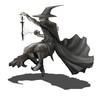## Welcome to the Treehouse Community

Want to collaborate on code errors? Have bugs you need feedback on? Looking for an extra set of eyes on your latest project? Get support with fellow developers, designers, and programmers of all backgrounds and skill levels here with the Treehouse Community!

### Looking to learn something new?

Treehouse offers a seven day free trial for new students. Get access to thousands of hours of content and join thousands of Treehouse students and alumni in the community today.# can't move forward

under product do i multiply on that line or simply enter the total

types.swift
```// Enter your code below
let firstValue: Int = 10
let secondValue: Int = 10
let product: Int = 100
let output: String = "the product of first and second value is 100"
```Let me know if this works or not

/// I assume you want to multiply values here under product?

```let product: Int = firstvalue * secondvalue
```

///You want to use string interpolation to pass in a INT value inside a string

```let output:String = "The product of \(firstValue) times \(secondValue) is \(product)."
```